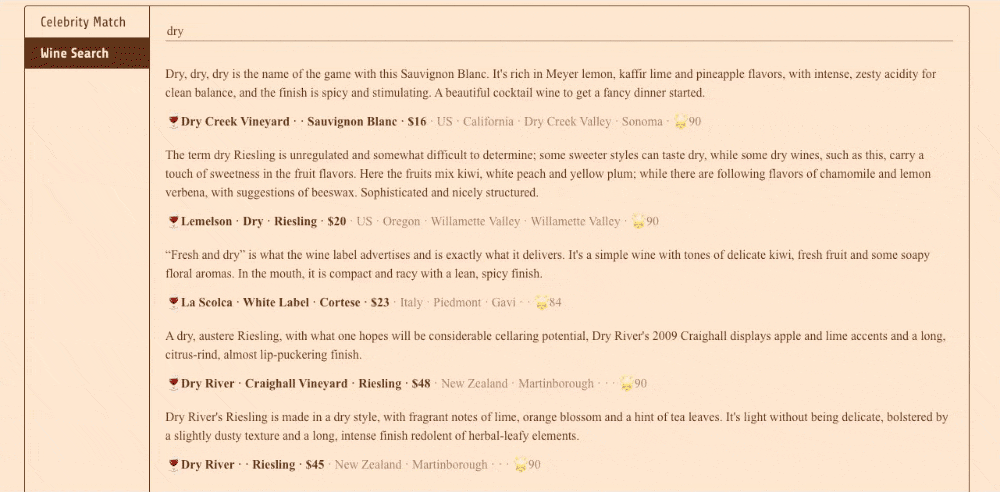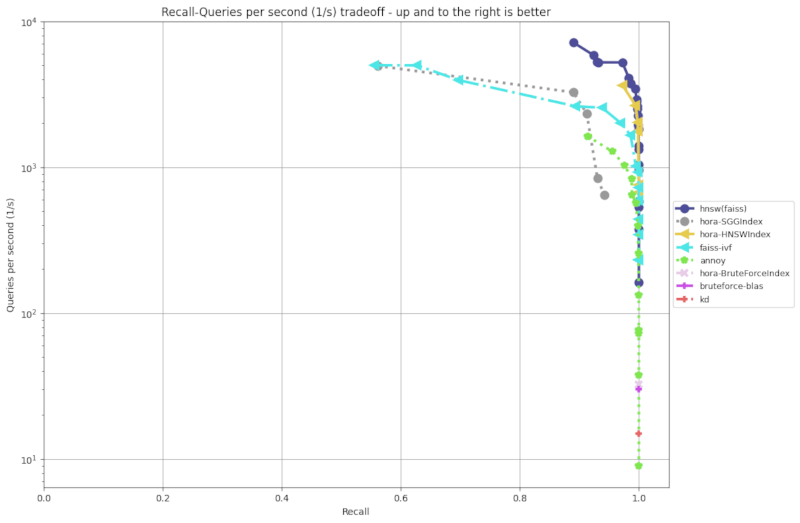## no-std hora

Hora Search Everywhere

### 2 releases

 0.1.1 Aug 7, 2021 Jul 24, 2021

#373 in Algorithms

Apache-2.0

9.5MB
4K SLoC# Hora

[Homepage] [Document] [Examples]

Hora Search Everywhere!

Hora is an approximate nearest neighbor search algorithm (wiki) library. We implement all code in `Rust🦀` for reliability, high level abstraction and high speeds comparable to `C++`.

Hora, `「ほら」` in Japanese, sounds like `[hōlə]`, and means `Wow`, `You see!` or `Look at that!`. The name is inspired by a famous Japanese song `「小さな恋のうた」`.

# Demos

👩 Face-Match [online demo], have a try!🍷 Dream wine comments search [online demo], have a try!# Features

• Performant ⚡️

• SIMD-Accelerated (packed_simd)
• Stable algorithm implementation
• Supports Multiple Languages ☄️

• `Python`
• `Javascript`
• `Java`
• `Go` (WIP)
• `Ruby` (WIP)
• `Swift` (WIP)
• `R` (WIP)
• `Julia` (WIP)
• Can also be used as a service
• Supports Multiple Indexes 🚀

• `Hierarchical Navigable Small World Graph Index (HNSWIndex)` (details)
• `Satellite System Graph (SSGIndex)` (details)
• `Product Quantization Inverted File(PQIVFIndex)` (details)
• `Random Projection Tree(RPTIndex)` (LSH, WIP)
• `BruteForce (BruteForceIndex)` (naive implementation with SIMD)
• Portable 💼

• Supports `WebAssembly`
• Supports `Windows`, `Linux` and `OS X`
• Supports `IOS` and `Android` (WIP)
• Supports `no_std` (WIP, partial)
• No heavy dependencies, such as `BLAS`
• Reliability 🔒

• `Rust` compiler secures all code
• Memory managed by `Rust` for all language libraries such as `Python's`
• Supports Multiple Distances 🧮

• `Dot Product Distance`
• `Euclidean Distance`
• `Manhattan Distance`
• `Cosine Similarity`
• Productive

• Well documented
• Elegant, simple and easy to learn API

# Installation

`Rust`

in `Cargo.toml`

``````[dependencies]
hora = "0.1.1"
``````

`Python`

``````\$ pip install horapy
``````

`Javascript (WebAssembly)`

``````\$ npm i horajs
``````

`Building from source`

``````\$ git clone https://github.com/hora-search/hora
\$ cargo build
``````

# Benchmarksby `aws t2.medium (CPU: Intel(R) Xeon(R) CPU E5-2686 v4 @ 2.30GHz)` more information

# Examples

`Rust` example [more info]

``````use hora::core::ann_index::ANNIndex;
use rand_distr::{Distribution, Normal};

pub fn demo() {
let n = 1000;
let dimension = 64;

// make sample points
let mut samples = Vec::with_capacity(n);
let normal = Normal::new(0.0, 10.0).unwrap();
for _i in 0..n {
let mut sample = Vec::with_capacity(dimension);
for _j in 0..dimension {
}
samples.push(sample);
}

// init index
let mut index = hora::index::hnsw_idx::HNSWIndex::<f32, usize>::new(
dimension,
&hora::index::hnsw_params::HNSWParams::<f32>::default(),
);
for (i, sample) in samples.iter().enumerate().take(n) {
}
index.build(hora::core::metrics::Metric::Euclidean).unwrap();

let target: usize = rng.gen_range(0..n);
// 523 has neighbors: [523, 762, 364, 268, 561, 231, 380, 817, 331, 246]
println!(
"{:?} has neighbors: {:?}",
target,
index.search(&samples[target], 10) // search for k nearest neighbors
);
}
``````

`Python` example [more info]

``````import numpy as np
from horapy import HNSWIndex

dimension = 50
n = 1000

# init index instance
index = HNSWIndex(dimension, "usize")

samples = np.float32(np.random.rand(n, dimension))
for i in range(0, len(samples)):

index.build("euclidean")  # build index

target = np.random.randint(0, n)
# 410 in Hora ANNIndex <HNSWIndexUsize> (dimension: 50, dtype: usize, max_item: 1000000, n_neigh: 32, n_neigh0: 64, ef_build: 20, ef_search: 500, has_deletion: False)
# has neighbors: [410, 736, 65, 36, 631, 83, 111, 254, 990, 161]
print("{} in {} \nhas neighbors: {}".format(
target, index, index.search(samples[target], 10)))  # search

``````

`JavaScript` example [more info]

``````import * as horajs from "horajs";

const demo = () => {
const dimension = 50;
var bf_idx = horajs.BruteForceIndexUsize.new(dimension);
// var hnsw_idx = horajs.HNSWIndexUsize.new(dimension, 1000000, 32, 64, 20, 500, 16, false);
for (var i = 0; i < 1000; i++) {
var feature = [];
for (var j = 0; j < dimension; j++) {
feature.push(Math.random());
}
}
bf_idx.build("euclidean"); // build index
var feature = [];
for (var j = 0; j < dimension; j++) {
feature.push(Math.random());
}
console.log("bf result", bf_idx.search(feature, 10)); //bf result Uint32Array(10) [704, 113, 358, 835, 408, 379, 117, 414, 808, 826]
}

(async () => {
await horajs.default();
await horajs.init_env();
demo();
})();
``````

`Java` example [more info]

``````public void demo() {
final int dimension = 2;
final float variance = 2.0f;
Random fRandom = new Random();

BruteForceIndex bruteforce_idx = new BruteForceIndex(dimension); // init index instance

List<float[]> tmp = new ArrayList<>();
for (int i = 0; i < 5; i++) {
for (int p = 0; p < 10; p++) {
float[] features = new float[dimension];
for (int j = 0; j < dimension; j++) {
features[j] = getGaussian(fRandom, (float) (i * 10), variance);
}
}
}
bruteforce_idx.build("bf", "euclidean"); // build index

int search_index = fRandom.nextInt(tmp.size());
// nearest neighbor search
int[] result = bruteforce_idx.search("bf", 10, tmp.get(search_index));
// [main] INFO com.hora.app.ANNIndexTest  - demo bruteforce_idx[7, 8, 0, 5, 3, 9, 1, 6, 4, 2]
log.info("demo bruteforce_idx" + Arrays.toString(result));
}

private static float getGaussian(Random fRandom, float aMean, float variance) {
float r = (float) fRandom.nextGaussian();
return aMean + r * variance;
}
``````

• Full test coverage
• Implement EFANNA algorithm to achieve faster KNN graph building
• Swift support and iOS/macOS deployment example
• Support `R`
• support `mmap`

# Related Projects and Comparison

• `Hora`'s implementation is strongly inspired by these libraries.
• `Faiss` focuses more on the GPU scenerio, and `Hora` is lighter than Faiss (no heavy dependencies).
• `Hora` expects to support more languages, and everything related to performance will be implemented by Rust🦀.
• `Annoy` only supports the `LSH (Random Projection)` algorithm.
• `ScaNN` and `Faiss` are less user-friendly, (e.g. lack of documentation).
• Hora is ALL IN RUST 🦀.
• `Milvus` and `Vald` also support multiple languages, but serve as a service instead of a library
• `Milvus` is built upon some libraries such as `Faiss`, while `Hora` is a library with all the algorithms implemented itself

# Contribute

We are glad to have you participate, any contributions are welcome, including documentations and tests. You can create a `Pull Request` or `Issue` on GitHub, and we will review it as soon as possible.

We use GitHub issues for tracking suggestions and bugs.

#### Clone the repo

``````git clone https://github.com/hora-search/hora
``````

#### Build

``````cargo build
``````

#### Test

``````cargo test --lib
``````

#### Try the changes

``````cd examples
cargo run
``````